Courses

# Floating Point Representation Computer Science Engineering (CSE) Notes | EduRev

## Computer Science Engineering (CSE) : Floating Point Representation Computer Science Engineering (CSE) Notes | EduRev

The document Floating Point Representation Computer Science Engineering (CSE) Notes | EduRev is a part of Computer Science Engineering (CSE) category.
All you need of Computer Science Engineering (CSE) at this link: Computer Science Engineering (CSE)

Floating Point Representation
The floating point representation of the number has two parts. The first part represents a signed fixed point numbers called mantissa or significand. The second part designates the position of the decimal (or binary) point and is called exponent. For example, the decimal no + 6132.789 is represented in floating point with fraction and exponent as follows.

Fraction                   Exponent
+0.6132789                +04

This representation is equivalent to the scientific notation +0.6132789 × 10+4 The floating point is always interpreted to represent a number in the following form ±M × R±E.
Only the mantissa M and the exponent E are physically represented in the register (including their sign). The radix R and the radix point position of the mantissa are always assumed.

A floating point binary no is represented in similar manner except that it uses base 2 for the exponent.

For example, the binary no +1001.11 is represented with 8 bit fraction and 0 bit exponent as follows.

0.1001110 × 2100

Fraction            Exponent
01001110           000100

The fraction has zero in the leftmost position to denote positive. The floating point number is equivalent to M × 2E = +(0.1001110)2 × 2+4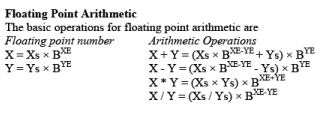There are four basic operations for floating point arithmetic. For addition and subtraction, it is necessary to ensure that both operands have the same exponent values. This may require shifting the radix point on one of the operands to achieve alignment. Multiplication and division are straighter forward.

A floating point operation may produce one of these conditions:

• Exponent Overflow: A positive exponent exceeds the maximum possible exponent value.
• Exponent Underflow: A negative exponent which is less than the minimum possible value.
• Significand Overflow: The addition of two significands of the same sign may carry in a carry out of the most significant bit.
• Significand underflow: In the process of aligning significands, digits may flow off the right end of the significand.

In floating point arithmetic, addition and subtraction are more complex than multiplication and division. This is because of the need for alignment. There are four phases for the algorithm for floating point addition and subtraction.
1. Check for zeros: Because addition and subtraction are identical except for a sign change, the process begins by changing the sign of the subtrahend if it is a subtraction operation. Next; if one is zero, second is result.
2. Align the Significands: Alignment may be achieved by shifting either the smaller number to the right (increasing exponent) or shifting the large number to the left (decreasing exponent).
3. Addition or subtraction of the significands: The aligned significands are then operated as required.
4. Normalization of the result: Normalization consists of shifting significand digits left until the most significant bit is nonzero.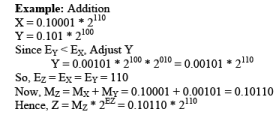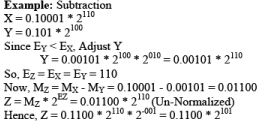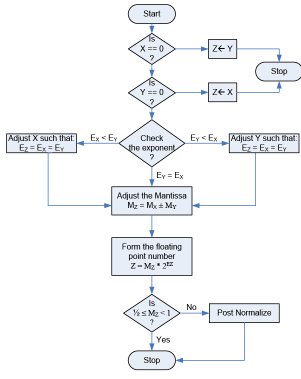Floating Point Multiplication
The multiplication can be subdivided into 4 parts.
1. Check for zeroes.
3. Multiply mantissa.
4. Normalize the product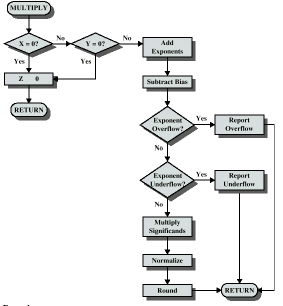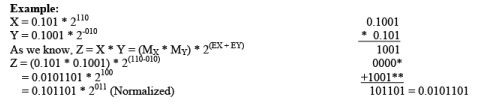Floating Point Division
The division algorithm can be subdivided into 5 parts
1. Check for zeroes.
2. Initial registers and evaluates the sign.
3. Align the dividend.
4. Subtract the exponent.
5. Divide the mantissa.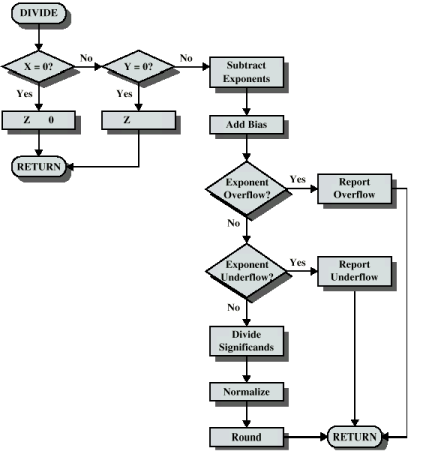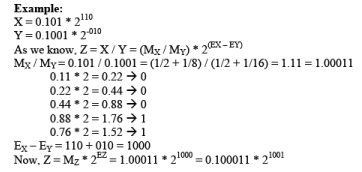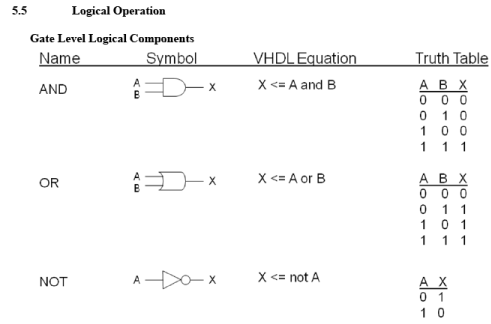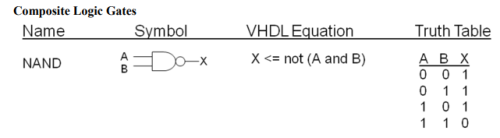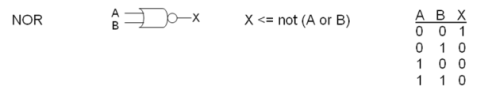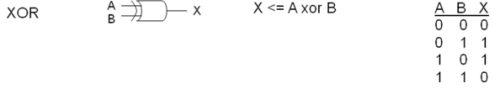Offer running on EduRev: Apply code STAYHOME200 to get INR 200 off on our premium plan EduRev Infinity!

,

,

,

,

,

,

,

,

,

,

,

,

,

,

,

,

,

,

,

,

,

;